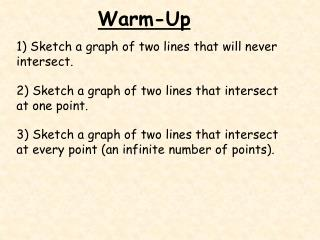Download PresentationWarm-Up# Warm-Up - PowerPoint PPT Presentation

Download Presentation##### Warm-Up

Download Policy: Content on the Website is provided to you AS IS for your information and personal use and may not be sold / licensed / shared on other websites without getting consent from its author. While downloading, if for some reason you are not able to download a presentation, the publisher may have deleted the file from their server.

- - - - - - - - - - - - - - - - - - - - - - - - - - - E N D - - - - - - - - - - - - - - - - - - - - - - - - - - -
##### Presentation Transcript

1. Warm-Up 1) Sketch a graph of two lines that will never intersect. 2) Sketch a graph of two lines that intersect at one point. 3) Sketch a graph of two lines that intersect at every point (an infinite number of points).

2. B10Solving Systems of Equations by Graphing Objectives: To find the solution of a system of equations by graphing

3. Activity Part 1 - Graph the equation 2x + 3y = 12 Part 2 - Graph the equation x – 4y = -5 How many points of intersection are there? 1

4. Activity Part 1 - Graph the equation x = 2y + 1 Part 2 - Graph the equation 3x – 6y = 9 How many points of intersection are there? 0

5. Activity Part 1 - Graph the equation 2x = 4 - y Part 2 - Graph the equation 6x + 3y = 12 How many points of intersection are there? an infinite number

6. 8 6 4 2 6 8 -2 -8 -6 -4 2 4 -2 -4 -6 -8 Example 1 Solve the following system by graphing. x + y = 2 x = y x + y = 2 0 2 1 1 5 -3 (1,1) x = y 0 0 1 1 5 5

7. Practice Solve by graphing. • x + 4y = -6 • 2x – 3y = -1 • y + 2x = 5 • 2y – 5x = 10

8. 4 minutes Warm-Up Solve by graphing. 1) y – 2x = 7 y = 2x + 8 2) 3y – 2x = 6 4x – 6y = -12

9. Example 1 Determine whether (3,5) is a solution of the system. y = 4x - 7 x + y = 8 5 = 4( ) - 7 3 3 + 5 = 8 5 = 12 - 7 8 = 8 5 = 5 (3,5) is a solution of the system

10. Example 2 Determine whether (-2,1) is a solution of the system. 2x – y = -5 3x + 2y = 3 2( ) -2 = -5 - 1 3( ) + 2( ) 1 = 3 -2 -4 – 1 = -5 -6 + 2 = 3 -5 = -5 (-2,1) is not a solution of the system

11. Practice Determine whether the given ordered pair is a solution of the system. • (2,-3); x = 2y + 8 • 2x + y = 1 • (-3,4); 2x = -y – 2 • y = -4

12. Practice Solve these systems by graphing. • x + 4y = -6 • 2x – 3y = -1 • y + 2x = 5 • 2y – 5x = 10